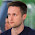## вторник, 14 января 2014 г.

### Codility. Train. Brackets ★

A string S consisting of N characters is considered to be properly nested if any of the following conditions is true:
• S is empty;
• S has the form "(U)" or "[U]" or "{U}" where U is a properly nested string;
• S has the form "VW" where V and W are properly nested strings.
For example, the string "{[()()]}" is properly nested but "([)()]" is not.
Write a function:
int solution(char *S);
that, given a string S consisting of N characters, returns 1 if S is properly nested and 0 otherwise.
For example, given S = "{[()()]}", the function should return 1 and given S = "([)()]", the function should return 0, as explained above.
Assume that:
• N is an integer within the range [0..200,000];
• string S consists only of the following characters: "(", "{", "[", "]", "}" and/or ")".
Complexity:
• expected worst-case time complexity is O(N);
• expected worst-case space complexity is O(N) (not counting the storage required for input arguments).

Solution:
```from string import maketrans

def solution(S):
b, t = [], maketrans('}])','{[(')
for i in S:
if i in '{[(':
b.append(i); continue
if len(b) == 0 or \
i.translate(t) != b.pop():
return 0
return 1 if len(b) == 0 else 0
```

#### 1 комментарий:

1.Здравствуйте. У меня есть задачки кодилити которых нет в этом блоге и которые было бы интересно осветить. Если интересно дайте знать вышлю вам мое мыло menvil.menvil@gmail.com